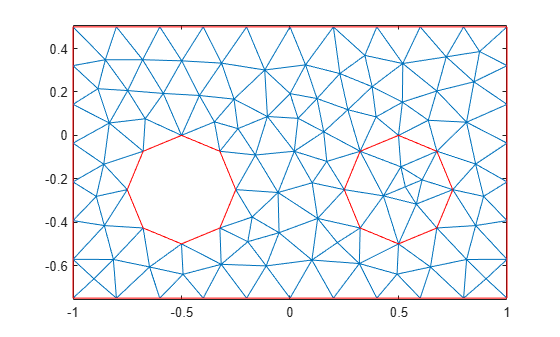pdesde

Indices of edges of mesh elements belonging to the specified faces of 2-D geometry

pdesde is not recommended. Use faceEdges instead.

Description

example

i = pdesde(e,FaceID) returns the indices of the [p,e,t] mesh edges that belong to outer boundaries of the geometry for a set of faces listed in FaceID.

i = pdesde(e) assumes that FaceID is a list of all faces of a 2-D geometry.

Examples

collapse all

Find the indices of the mesh elements' edges located on the outer boundaries of the geometry and belonging to the specified faces.

Define two circles and a rectangle and place these in one matrix.

R1 = [3,4,-1,1,1,-1,0.5,0.5,-0.75,-0.75]';
C1 = [1,-0.5,-0.25,0.25]';
C2 = [1,0.5,-0.25,0.25]';
C1 = [C1;zeros(length(R1) - length(C1),1)];
C2 = [C2;zeros(length(R1) - length(C2),1)];
gd = [R1,C1,C2];

Create a set formula that subtracts one circle from the rectangle and adds the other circle to the rectangle.

sf = 'R1-C1+C2';

Create and plot the geometry.

ns = char('R1','C1','C2');
ns = ns';
gd = decsg(gd,sf,ns);
pdegplot(gd,'FaceLabels','on')Create and plot a mesh.

[p,e,t] = initmesh(gd);
pdemesh(p,e,t)Find the indices of the mesh elements' edges located on the outer boundaries and belonging to face 1. Display the result as a column vector.

i1 = pdesde(e,1);
i1.'
ans = 42×1

1
2
3
4
5
6
7
8
9
10
⋮

The resulting vector contains indices of all mesh edges in this geometry, except the eight internal edges surrounding face 2.

length(e) - length(i1)
ans = 8

Use the pdesde function to find the mesh edges surrounding face 2. The result is an empty vector because none of these mesh edges belong to the outer boundary of the geometry.

i2 = pdesde(e,2)
i2 =

1x0 empty double row vector

Input Arguments

collapse all

Mesh edges, specified as a 7-by-Ne matrix of edges, where Ne is the number of edges in the mesh. For details on the mesh data representation, see initmesh.

Data Types: double

Face IDs, specified as a vector of integers.

Data Types: double

Output Arguments

collapse all

Indices of the mesh edges on the outer boundaries, returned as a vector of integers.